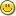### 1. Smallest Non-Zero Number from rnd()

What is the smallest non-zero number that rnd() will return?

It seems to be 0.01. If so, is there any way to make it more precise?

For example, to get numbers between 0.001 and 1? or 0.0001 and 1?

I guess I could always multiply to get integers and use rand_range()...

### 2. Re: Smallest Non-Zero Number from rnd()

euphoric said...

What is the smallest non-zero number that rnd() will return?

It seems to be 0.01. If so, is there any way to make it more precise?

For example, to get numbers between 0.001 and 1? or 0.0001 and 1?

I guess I could always multiply to get integers and use rand_range()...

Perhaps I'm misunderstanding the question, but a test shows me that rnd() can return numbers smaller than 0.01:

```include std/rand.e
atom x
while 1 do
x = rnd()
if x < 0.001 then ? x end if
end while
```

```0.0006218517863
0.0009995880243
0.0002405867837
0.000242709016
0.0004686160389
0.0007781575098
0.0003000935483
```

### 3. Re: Smallest Non-Zero Number from rnd()

irv said...
euphoric said...

What is the smallest non-zero number that rnd() will return?

Perhaps I'm misunderstanding the question, but a test shows me that rnd() can return numbers smaller than 0.01:

```include std/rand.e
atom x
while 1 do
x = rnd()
if x < 0.001 then ? x end if
end while
```

```0.0006218517863
0.0009995880243
0.0002405867837
0.000242709016
0.0004686160389
0.0007781575098
0.0003000935483
```

Oh, nice! I guess my testing wasn't thorough enough. I never saw any numbers that small.

The question remains, what is the smallest? I'd have to guess it's going to be as precise as the highest decimal point, so maybe 0.0000000000001?Although, from your test, it looks like 0.0001 will be the smallest.

ADD: Just did a test, and I'm getting numbers lower than 0.0000001! Wowie!

### 4. Re: Smallest Non-Zero Number from rnd()

Just keep adding zeros to the test. It appears that the number below is a practical limit - if not an absolute limit. Reason being, it takes longer and longer to get 'hits' that fall into the < 0.00000001 limit - most results are larger than the limit, and so are discarded.

```if x < 0.00000001 then printf(1,"%.12f\n",x) end if
```

```0.000000007340
0.000000003116
0.000000005185
0.000000008549
0.000000002161
0.000000003722

```